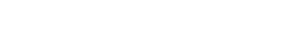Drag

# Matlab生物代写｜EBME 359 Lab 4: Neural Modeling Part 1

## BACKGROUND:

An axon is made up of sequential Nodes of Ranvier1 separated by myelinated “internodes.” In this lab, you will
learn about the two of the ion channels that exist in a Node of Ranvier. These ion channels are what are responsible for
forming the shape of the action potential. An individual Node of Ranvier is represented by the model below. Note, often
you will see Rx expressed as Gx, the conductance of ion x. Rx=1/Gx. This is the Hodgkin Huxley model of a Node of
Ranvier and it’s based on the squid giant axon.I current through entire Node of Ranvier
IC capacitive current
INa sodium ion current
IK potassium ion current
IL “leakage” (non-specific) ionic current
𝐶𝐶𝑚𝑚 membrane capacitance
RNa variable resistance to sodium flow
RK variable resistance to potassium flow
RL fixed resistance to leakage flow
ENa reversal potential for sodium
EK reversal potential for potassium
EL reversal potential for leakage current.

The analysis of the circuit yields the following:Where 𝑔𝑔̅𝑥𝑥 (𝑚𝑚𝑚𝑚/𝑐𝑐𝑚𝑚2) is the maximum conductance for ion x (Sodium, potassium, or
non-specific leakage), Ex is the reversal potential of ion x, and Vm is the voltage across
the axon membrane. The reversal potential is the membrane voltage at which the ion in
question will start to flow the opposite direction (either into or out of the cell). The n, m,
and h terms describe the probability that an ion “gate” is open, allowing the ion to flow
across the membrane. Sodium ion gates are controlled by two different types of gates: m
and h. There are 3 m-gates and 1 h-gate per sodium channel. Each gate’s probability of
being open ranges from 0-1 (or 0%-100%). Thus, the total probability that a sodium
channel is open is the probability that each of the 4 gates are open, which is m3h.

Potassium gates are controlled by 4 n-gates. The rates at which ion gates open or close
are a function of both membrane voltage and time.

Solving for the derivative: